# HBSE 6th Class Maths Solutions Chapter 11 Algebra Ex 11.1

Haryana State Board HBSE 6th Class Maths Solutions Chapter 11 Algebra Ex 11.1 Textbook Exercise Questions and Answers.

## Haryana Board 6th Class Maths Solutions Chapter 11 Algebra Exercise 11.1

Question 1.
Find the rule, which gives the number of matchsticks required to make the following matchstick patterns. Use a variable to write the rule.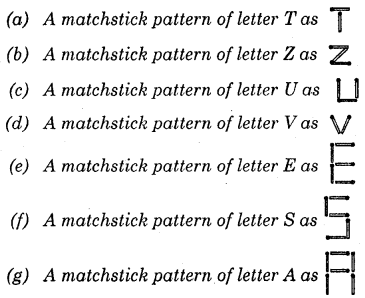Solution:
(a)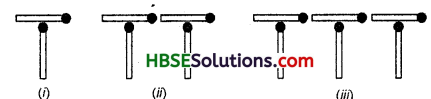To make one T, we use two matchsticks as shown in Fig.(i)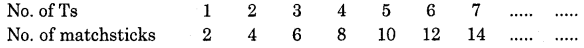Thus, number of matchsticks required = 2n; n is a variable taking values 1, 2, 3, 4 ………..

(b) To make one Z, we use three matchsticks as shown in Fig. 11.2(f)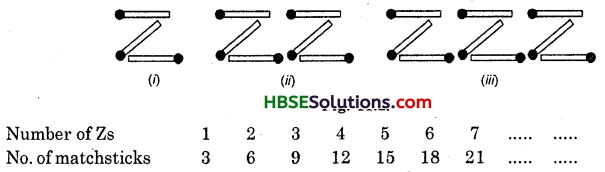Thus, number of matchsticks required = 3n; n is a variable taking values 1, 2, 3, ……………(c) To make one U, we use three matchsticks as shown in Fig. (i)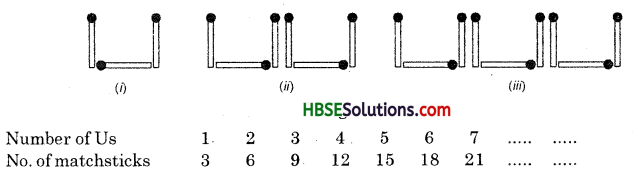Thus, number of matchsticks required = 3n; n is a variable taking values 1, 2, 3, 4,

(d) To make one V, we use two matchsticks as shown in Fig. (i)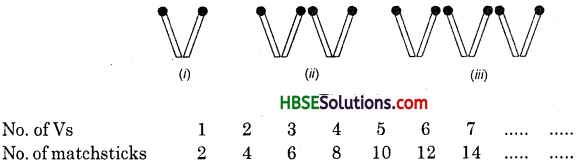Thus, number of matchsticks required = 2n; n is a variable taking values 1, 2, 3, 4, 5, ………….

(e) To make one E, we use five matchsticks as shown in Fig. (i)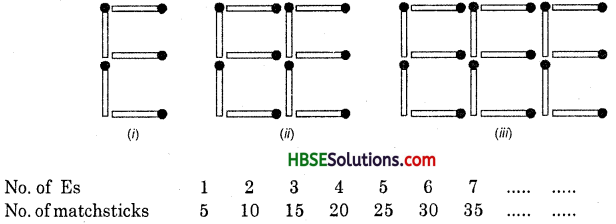Thus, number of matchsticks required = 5n; n is a variable taking values 1, 2, 3, …………

(f) To make one S, we use five matchsticks as shown in Fig. (i)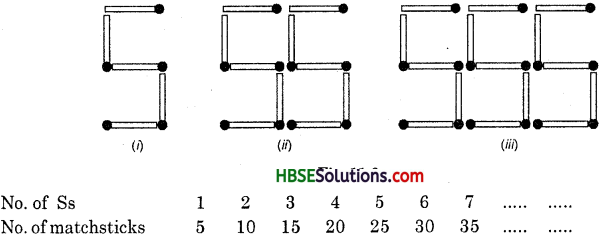Thus, number of matchsticks required = 5n; n is a variable taking values 1, 2, 3, 4, 5

(g) To make one A, we use six rnatchsticks as shown in Fig.(i)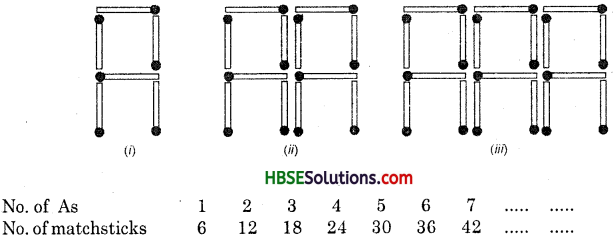Thus, number of matchsticks required = 6n; n is a variable taking values 1, 2, 3, 4, 5, …………….

Question 2.
We already know the rule for the pattern of letters L, C and F. Some of the letters from Question 1 (given back) give us the same rule as that given by L. Which are these ? Why does this happen ?
Solution:
(a) and (d) give us the same rule as that given by L. Because to make one T and one V, we require two matchsticks.Question 3.
Cadets are marching in a parade. There are 5 cadets in a row. What is the rule, which gives the number of cadets, given the number of rows ? (Use ‘n’ for the number of rows).
Solution:
The number of cadets will depend on the number of rows. If there is 1 row, there will be 5 cadets. If there are 2 rows, there will be 2 x 5 or 10 cadets and so on. If there are ‘n’ rows, there will be n x 5 or 5n cadets, here n is a variable which stands for the number of rows and takes values 1, 2, 3, 4 ……..

Question 4.
If there are 50 mangoes in a box, how will you write the total number of mangoes in terms of the number of boxes ? (Use ‘6’ for the number of boxes.)
Solution:
Number of mangoes in one box = 50
Number of mangoes in two boxes = 50 x 2 = 100
Number of mangoes in three boxes
= 50 x 3 = 150 and so on.
Number of mangoes in ‘b’ boxes = 50 x b = 50b
where ‘b’ is the no. of boxes.

Question 5.
The teacher distributes 5 pencils per student. Can you tell how many pencils are needed, given the number of
students ? (Use ‘s’ for the number of students.)
Solution:
Number of pencils needed for one student = 5
Number of pencils needed for two students = 5 x 2 = 10
Number of pencils needed for three students
= 5 x 3 = 15 and so on.
∴ Number of pencils needed for ‘s’ students = 5 x s = 50s.
where ‘s’ is the number of students.

Question 6.
A bird flies 1 kilometre in one minute. Can you express the distance covered by the bird in terms of its flying time in minutes ? (Use T for flying time in minutes.)
Sol. Distance covered by the bird in one minute = 1 km
Distance covered by the bird in two minutes = 1 x 2 = 2 km
Distance covered by the bird in three minutes
= 1 x 3 = 3 Ion and so on.
∴ Distance covered by the bird in‘t’ minutes 1 x t = t km.
where ‘t’ is the flying time in minutes.Question 7.
Radha is drawing a dot Rangoli (a beautiful pattern of lines joining dots with chalk powder as in (Fig. 11.8). She has 8 dots in a row. How many dots will her Rangoli have for V rows ? How many dots are there if there are 8 rows ? If there are 10 rows ?
Solution: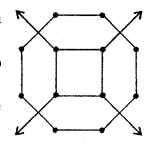Number of dots in one row = 8
Number of dots in two rows = 8 x 2 = 16
Number of dots in three rows = 8 x 3 = 24 and so on.
Number of dots in rows = 8 x r = 8r
Now, number of dots in 8 rows = 8 x 8 = 64
and number of dots in 10 rows = 8 x 10 = 80

Question 8.
Leela is Radha’s younger sister. Leela is 4 years younger than Radha. Can you write Leela’s age in terms of Radha’s age ? Take Radha’s age to be V years.
Solution:
∵ Leela is 4 years younger than Radha.
∴ Leela’s age will be 4 years less than Radha’s age.
If Radha’s age = x years, then Leela’s age = (x – 4) years.

Question 9.
Mother has made laddus. She gives some laddus to guests and family members, still 5 laddus remain. If the number of laddus mother gave away is ‘l’, how many laddus did she make ?
Solution:
Number of laddus given to guests and family members = l
Number of laddus still remain = 5

Question 10.
Oranges are to be transferred from larger boxes into smaller boxes. When a large box is emptied, the oranges from it fill two smaller boxes and still 10 oranges remain outside. If the number of oranges in a small box are taken to be x, what is the number of oranges in the larger box ?
Solution:
Number of oranges in one small box = x
Number of oranges in two small boxes = 2x
Number of oranges remain outside = 10
∴ Number of oranges in the larger box = 2x + 10.

Question 11.
(a) Look at the following matchstick pattern of squares (Fig. 11.9).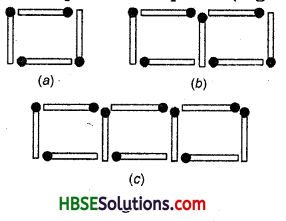The squares are not separate. Two neighbouring squares have a common matchstick. Observe the pattern and find the rule that gives the number of matchsticks in terms of the number of squares.
(Hint: If you remove the vertical stick at the end, you will get a pattern of Cs.)
Solution: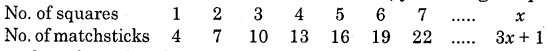Thus, number of matchsticks required = 3x + 1, where x is a variable and represents the number of squares.(b) Fig. 11.10 gives a matchstick pattern of triangles. As in Exercise 11(a) above, find the general rule that gives the no. of matchsticks in terms of the number of triangles.Solution: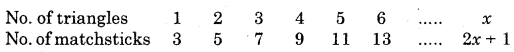Thus number of matchsticks required = 2x + 1, where x is a variable and represents the number of triangles.